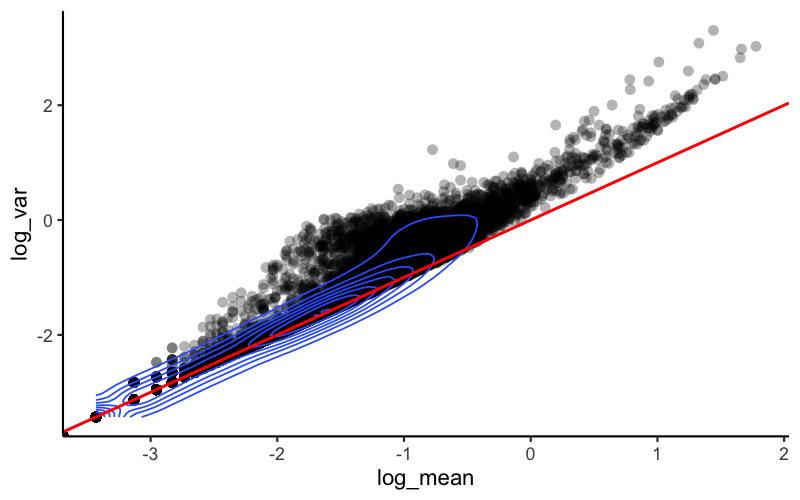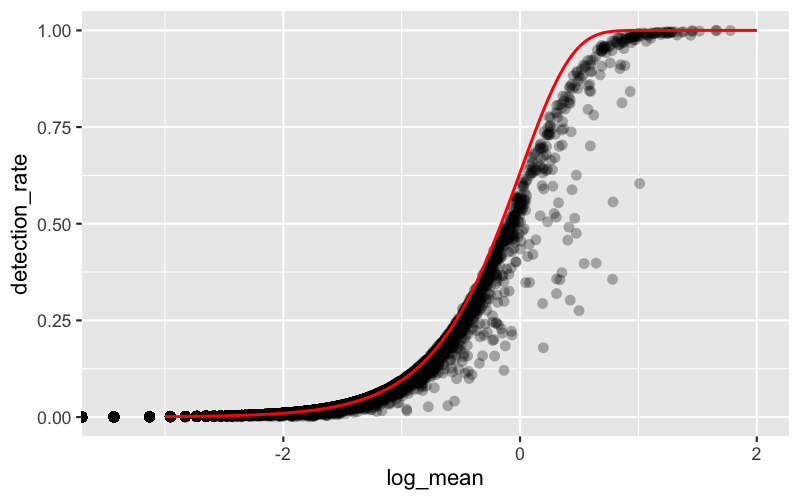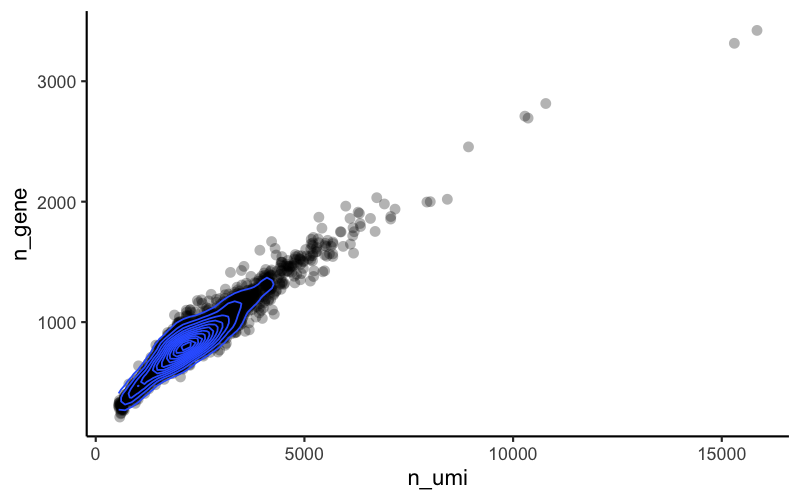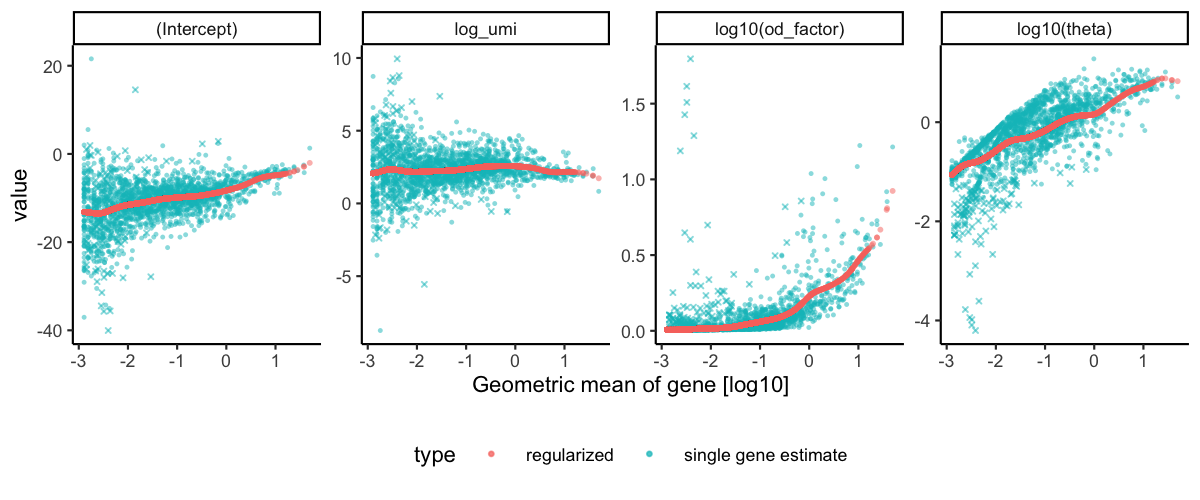With this vignette we introduce the concept of using regularized regression for normalization and show some examples.

First we will follow the Seurat clustering tutorial and load a dataset of Peripheral Blood Mononuclear Cells (PBMC) freely available from 10X Genomics. There are 2,700 single cells that were sequenced on the Illumina NextSeq 500. The raw data can be found here.

pbmc_data <- readRDS(file = "~/Projects/data/pbmc3k_umi_counts.Rds")
class(pbmc_data)
#>  "dgCMatrix"
#> attr(,"package")
#>  "Matrix"
dim(pbmc_data)
#>  32738  2700

pbmc_data is a sparse matrix of UMI counts (32,738 genes as rows and 2,700 cells as columns).

### Inspect data

We will now calculate some properties and visually inspect the data. Our main interest is in the general trends not in individual outliers. Neither genes nor cells that stand out are important at this step, instead we want to focus on the global trends.

Derive gene and cell attributes from the UMI matrix.

gene_attr <- data.frame(mean = rowMeans(pbmc_data), detection_rate = rowMeans(pbmc_data >
0), var = apply(pbmc_data, 1, var))
gene_attr$log_mean <- log10(gene_attr$mean)
gene_attr$log_var <- log10(gene_attr$var)
rownames(gene_attr) <- rownames(pbmc_data)
cell_attr <- data.frame(n_umi = colSums(pbmc_data), n_gene = colSums(pbmc_data >
0))
rownames(cell_attr) <- colnames(pbmc_data)
ggplot(gene_attr, aes(log_mean, log_var)) + geom_point(alpha = 0.3, shape = 16) +
geom_density_2d(size = 0.3) + geom_abline(intercept = 0, slope = 1, color = "red")Mean-variance relationship

For the genes, we can see that up to a mean UMI count of ca. 0.1 the variance follows the line through the origin with slop one, i.e. variance and mean are roughly equal as expected under a Poisson model. However, genes with a higher average UMI count show overdispersion compared to Poisson.

# add the expected detection rate under Poisson model
x = seq(from = -3, to = 2, length.out = 1000)
poisson_model <- data.frame(log_mean = x, detection_rate = 1 - dpois(0, lambda = 10^x))
ggplot(gene_attr, aes(log_mean, detection_rate)) + geom_point(alpha = 0.3, shape = 16) +
geom_line(data = poisson_model, color = "red") + theme_gray(base_size = 8)Mean-detection-rate relationship

In line with the previous plot, we see a lower than expected detection rate in the medium expression range. However, for the highly expressed genes, the rate is at or very close to 1.0 suggesting that there is no zero-inflation in the counts for those genes and that zero-inflation is a result of overdispersion, rather than an independent systematic bias.

ggplot(cell_attr, aes(n_umi, n_gene)) + geom_point(alpha = 0.3, shape = 16) + geom_density_2d(size = 0.3)UMI detected genes relationship

The more UMI counts a cell has, the more genes are detected. In this data set this seems to be an almost linear relationship (at least within the UMI range of most of the cells).

### General idea of transformation

Based on the observations above, which are not unique to this particular data set, we propose to model the expression of each gene as a negative binomial random variable with a mean that depends on other variables. Here the other variables can be used to model the differences in sequencing depth between cells and are used as independent variables in a regression model. In order to avoid overfitting, we will first fit model parameters per gene, and then use the relationship between gene mean and parameter values to fit parameters, thereby combining information across genes. Given the fitted model parameters, we transform each observed UMI count into a Pearson residual which can be interpreted as the number of standard deviations an observed count is away from the expected mean. If the model accurately describes the mean-variance relationship and the dependency of mean and latent factors, then the result should have mean zero and a stable variance across the range of expression.

### Estimate model parameters and transform data

The vst function estimates model parameters and performs the variance stabilizing transformation. Here we use the log10 of the total UMI counts of a cell as variable for sequencing depth for each cell. After data transformation we plot the model parameters as a function of gene mean (geometric mean).

set.seed(44)
vst_out <- sctransform::vst(pbmc_data, latent_var = c("log_umi"), return_gene_attr = TRUE,
return_cell_attr = TRUE, verbosity = 1)
#> Calculating cell attributes from input UMI matrix: log_umi
#> Variance stabilizing transformation of count matrix of size 12572 by 2700
#> Model formula is y ~ log_umi
#> Get Negative Binomial regression parameters per gene
#> Using 2000 genes, 2700 cells
#> Found 120 outliers - those will be ignored in fitting/regularization step
#> Second step: Get residuals using fitted parameters for 12572 genes
#> Calculating gene attributes
#> Wall clock passed: Time difference of 17.82073 secs
sctransform::plot_model_pars(vst_out, show_theta = TRUE)Internally vst performs Poisson regression per gene with $$log(\mu) = \beta_0 + \beta_1 x$$, where $$x$$ is log_umi, the base 10 logarithm of the total number of UMI counts in each cell, and $$\mu$$ are the expected number of UMI counts of the given gene. The previous plot shows $$\beta_0$$ (Intercept), the $$\beta_1$$ coefficient log_umi, and the maximum likelihood estimate of the overdispersion parameter theta under the negative binomial model. Under the negative binomial model the variance of a gene depends on the expected UMI counts and theta: $$\mu + \frac{\mu^2}{\theta}$$. Also shown is the overdispersion factor (od_factor), defined as $$1 + \frac{m}{\theta}$$ where $$m$$ is the gene mean.

In a second step the regularized model parameters are used to turn observed UMI counts into Pearson residuals.

### Inspect model

We will look at several genes in more detail.

sctransform::plot_model(vst_out, pbmc_data, c("MALAT1", "RPL10", "FTL"), plot_residual = TRUE)